Courses

# Test: Mole Concept, Volumetric & Redox- 1

## 20 Questions MCQ Test Physical Chemistry | Test: Mole Concept, Volumetric & Redox- 1

Description
This mock test of Test: Mole Concept, Volumetric & Redox- 1 for Chemistry helps you for every Chemistry entrance exam. This contains 20 Multiple Choice Questions for Chemistry Test: Mole Concept, Volumetric & Redox- 1 (mcq) to study with solutions a complete question bank. The solved questions answers in this Test: Mole Concept, Volumetric & Redox- 1 quiz give you a good mix of easy questions and tough questions. Chemistry students definitely take this Test: Mole Concept, Volumetric & Redox- 1 exercise for a better result in the exam. You can find other Test: Mole Concept, Volumetric & Redox- 1 extra questions, long questions & short questions for Chemistry on EduRev as well by searching above.
QUESTION: 1

### 4.4g of CO2 and 2.24 litre of H2 at STP (273.15 K and 1 atm pressure) are mixed in a container. The total of molecules present in the container will be

Solution:

Number of moles of CO2 =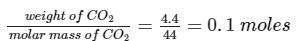22.4 L of H2 has 1 mole of H2
So ,number of moles in 2.24L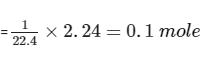Total moles is 0.2 moles.

Total molecules in 0.2moles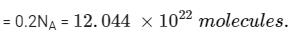QUESTION: 2

### 4I– + Hg2+ → HgI42– ; 1 mole of each Hg2+ and I– will form:

Solution:

Balanced reaction is as follows :

Hg2+(aq) + 4I- ​ (aq) ⇋​   HgI42-​ (aq)​

Thus
4 mole of I- produces =1 mole of HgI42-​​

1 mole I- produces =1/4 moles of HgI42-​​

QUESTION: 3

### How many gm of I2 are present in a solution which requires 40 ml of 0.11N Na2S2O3 to react with it via the reaction :  S2O32– + I2 → S4O62– + 2I– ?

Solution:

Meq.of I= Meq.of Na2S2O3
= 40×0.11
∴ w/254/2×1000
=40×0.11
wI2=0.558g

QUESTION: 4

Two elements A (atomic mass = 75) and B (atomic mass = 16) combine to yield a compound. The % by weight of A in the compound was found to be 75.08. The formula of compound is:

Solution:

Molecular weight of A2B3 = 75 × 2 + 16 × 3 = 198
198g of A2B3 =150g of A
∴ 10g of A2B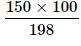= 75.08g of A

So option B is correct.

QUESTION: 5

What weight of HNO3 is needed to convert 5g of Iodine into Iodic acid according to the reaction:
I2 + HNO3→ HIO3 + NO2 +H2O

Solution:

The balanced reaction is:

I2 + 10HNO3 → 2HIO3 + 10NO2 + 4H2O

127*2g of iodine requires 63*10g of HNO3

Thus, 5g of HNO requires:

63*10/127*2 *5

= 12.g

QUESTION: 6

In the reaction, VO + Fe2O3 → FeO + V2O5, the  equilvalent weight of  V2O5 is equal to its

Solution: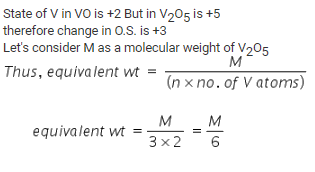QUESTION: 7

When BrO3 ion reacts with Br ion in acid solution Br2 is liberated. The eq. weight of KBrO3 in this reaction is:

Solution:

10e + 2Br5+ → Br02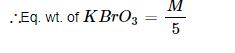QUESTION: 8

The hydrated salt, Na2SO4.nH2O, undergoes 55.9 % loss in weight on heating and become anhydrous. The value of n will be:

Solution:

Molecular Mass of Na2SO4 is =2 x 23 + 32 + 4 x 16 = 142 gm.

To know the mass of Na2SO4 containing 55.9% H2O , we subtract 55.9 from 100,  100 - 55.9 = 44.1

Therefore 44.1 g Na2SOcontains = 55.9 gm H2O

So, 1gm Na2SO4 contains = 55.9 / 44.1 gm H2O

142 gm Na2SO4 contains = 55.9 /44.1 x 142 = 180 gm H2O .

Number of water molecules = 180 / 18 (Since Molecular mass of H2O is 18).

= 10 water molecules .

Hence the value of n is 10 and the formula is Na2SO4.10H2O.

QUESTION: 9

When a metal is burnt, its weight is increased by 24%. The eq. weight of the metal will be:

Solution: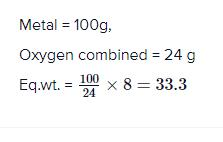QUESTION: 10

One mole of potassium chlorate is thermally decomposed and excess of aluminum is burnt in the gaseous product .How many moles of aluminum oxide are formed?

Solution:

2KClO→ 2KCl + 3O2

4Al + 3O→ 2Al2O3

The molar ratio for the first reaction is 2:3 for KClO3:O2

Thus, if 1 mole of  KClO3 is decomposed, 1.5 moles of O2 is produced.

The molar ratio for the second reaction is: 3:2 for O2:Al2O3

3 moles of oxygen produces 2 moles of aluminium oxide.

Since, we have 1.5 moles of oxygen, number of moles of Al2O3  produced =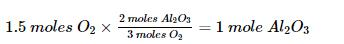QUESTION: 11

How much Cl2 at STP is liberated when one mole of KMnO4 reacts with HCl ?

Solution:

Potassium permanganate reacts with hydrochloric acid according the following equation:

2KMnO+ 16HCl → 2KCl + 2MnCl+ 8H2O + 5Cl2

2 moles of KMnO4 produces 5 moles of Cl2

1 mole of KMnO4 will produce 2.5 moles of Cl2

Volume of 2.5 moles of Cl= 56 litres

QUESTION: 12

Minimum quantity of H2S needed to precipitate 63.5g of Cu2+ is nearly:

Solution:

Chemical Reaction: Cu2++ H2S → CuS + 2H+

In this reaction, to precipitate 1 mol of Cu2+ we require 1 mol of H2S.

Moles of Cu2+ = 63.5 g / 63.5 g mol-1 = 1 mol

Hence, to precipitate 1 mol of Cu2+ we require 1 mol of H2S

Mass of H2S = 1 mol x 34 g mol-1 = 34 g

QUESTION: 13

2g of CaCO3 was treated with 0.1M HCl (500 ml). The volume of CO2 evolved at STP after heating the solution is:

Solution:
QUESTION: 14

10g of CaCO3 on heating gives 5g of the residue (as CaO). The % yield of the reaction is approx:

Solution:
QUESTION: 15

‘x’ g of KClO3 on decomposition gives ‘y’ ml of O2 at STP. The % purity of KClO3 would be

Solution:

The balanced eqn. will be
2KClO3 = 2KCl+3O2
the no. of moles of Oxygen = y/22.4

3 moles of Oxygen if produced from 2moles of KClO3 ...then 1 mole will produce from 2/3moles of KClO3 ...And y/22.4 will be producing from 2/3×y/22.4

,..no. of moles of KClO3 = 2/3×y/22.4
So..mass of KClO3 used =2/3×y/22.4×M(mol. wt.)

%purity = 2/3×y/22.4×M divide the whole to x
%purity=2×y×M/3×22.4×x

QUESTION: 16

33.6g of an impure sample of sodium bicarbonate when heated strongly gave 4.4g of CO2. The % purity of NaHCO3 would be:

Solution:

2NaHCO3 = Na2CO3 + CO2 + H2O
Moles of CO2 = 4.4/44 =0.1
Then moles of NaHCO3 =0.1×2/1 =0.2
And wt. Of NaHCO3 = 0.2 × 84 = 16.8gm
%purity of NaHCO3 = 16.8 × 100/33.6 =50%.

QUESTION: 17

0.16g of dibasic acid required 25ml of N/10 NaOH for complete neutralization. Molecular wt. of acid is:

Solution:

Dibasic acid NaOH;

N1V= N2V2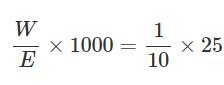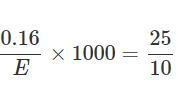M = 2 × E = 2 × 64 = 128

.

QUESTION: 18

The molar coefficients of AsO33– and MnO4 in the reaction are:

AsO33– + MnO4Θ → AsO43– + MnO2

Solution: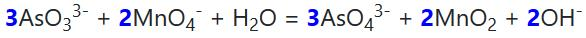QUESTION: 19

Which of the following is a disproportionation reaction?

Solution:

A disproportionation reaction is a reaction in which a single element undergoes reduction and oxidation i.e in option d),in Cu2O, Cu has an oxidation state of +1 and in Cu it has 0 (i.e reduction)

and +1 to +2 (i.e oxidation)

QUESTION: 20

Hydrogen and oxygen combine to form H2O2 and H2O containing 5.93% and 11.2 % hydrogen respectively. The data illustrates:​

Solution:

For predicting the law of chemical combination, we have to have find out the ratio of masses in both the cases:
For that, let the total mass in each case be 100 grams.
For H2O2:
​​Mass of hydrogen = 5.93 gm
Mass of oxygen = 100-5.93 = 94.07 gm
Ratio of mass of hydrogen to mass of oxygen,
i.e.      H:O = 5.93 : 94.07
H:O = 1 : 16
For H2O:
​Mass of hydrogen = 11.2 gm
Mass of oxygen = 100-11.2 = 88.8 gm
Ratio of mass of hydrogen to mass of oxygen,
i.e.     H:O = 11.2 : 88.8
H:O = 1 : 32
Now,
As the ratio of mass of hydrogen remains same in both the cases and the ratio of masses of oxygen in both the cases (16 : 32 or 1 : 2) is a whole number ratio.
Therefore, the above data illustrates the law of multiple proportions.
Hence, option (4) is correct.